# NVIDIA CUDA核心GPU實做：Jetson Nano 運用TensorRT加速引擎 – 上篇

*本文由RS components 贊助發表，轉載自DesignSpark部落格原文連結

# 目錄

• TensorRT
• TensorRT 的優化方式
• TensorRT vs Tensorflow Lite
• TensorRT建構流程
• ONNX (Open Neural Network Exchange)
• Jetson Nano安裝TensorRT.
• PyTorch 匯出 ONNX.
• 透過ONNX RUNTIME運行ONNX Model
• 使用TensorRT運行ONNX
• PyTorch使用TensorRT最簡單的方式
• YOLOv5使用TensorRT引擎方式
• 結語

# TensorRT

TensorRT是由 NVIDIA 所推出的深度學習加速引擎 （以下簡稱trt ），主要的目的是用在加速深度學習的 Inference，按照官方提出TensorRT比CPU執行快40倍的意思，就像是YOLOv5針對一張圖片進行推論用CPU的話大概是1秒，如果用上TensorRT的話可能就只要0.025秒而已，這種加速是非常明顯的！而這種加速大部分都是運用的邊緣裝置上 ( Edge Device )，由於邊緣裝置上的效能沒有一般電腦來的好，所以往往需要這種軟體加速。

# TensorRT 的優化方式

TensorRT主要的優化過程有這些： Precision Calibration、Layer & Tensor Fusion、Kernel Auto-Tuning、Dynamic Tensor Memory、Multi-Stream Execution，今天會介紹前兩種，後面三種比較底層資訊也比較少所以就先不介紹了。

### 對模型進行重構與優化（Layer & Tensor Fusion）

1.第一步先刪除沒有使用到的輸出層

2.垂直優化：由於CUDA會在每一層輸入的時候啟動，而這個垂直優化就是透過將常使用的層作合併，像圖中將Conv、bias、relu合併為CBR，減少CUDA啟動關閉的次數來達到時間上的縮減。

3.水平優化：理論同上，如果同層其他分支也在運作一樣的架構則直接合併，要注意的地方是合併成同一層之後輸出必須要做分割，再輸出到不同層去。

# TensorRT建構流程

1. ONNX parser：將模型轉換成ONNX的模型格式。
2. Builder：將模型導入TensorRT並且建構TensorRT的引擎。
3. Engine：引擎會接收輸入值並且進行Inference跟輸出。
4. Logger：負責記錄用的，會接收各種引擎在Inference時的訊息。

# Jetson Nano安裝TensorRT

#### 1.  官方作法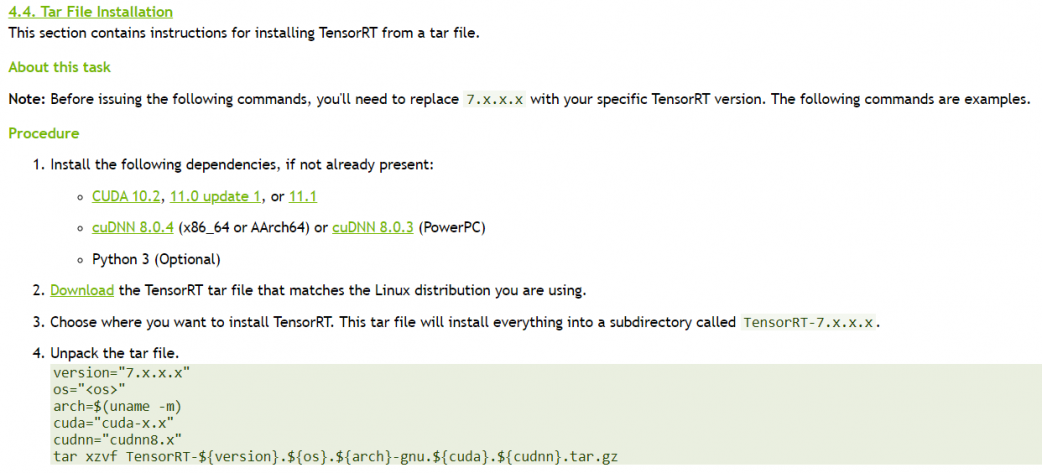https://docs.nvidia.com/deeplearning/tensorrt/install-guide/index.html#installing-tar

#### 2.  我的映像檔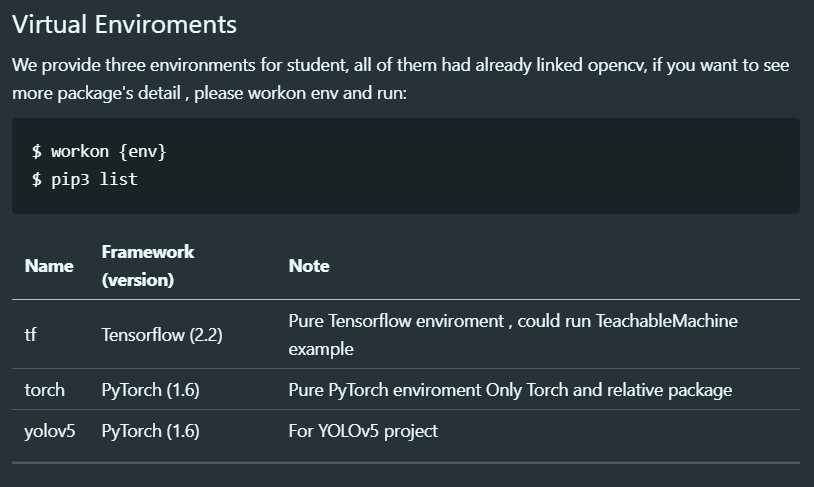### 將TensorRT連結到virtualenv

``\$ vim ~/.bashrcexport CUDA_HOME=/usr/local/cudaexport PATH=\$CUDA_HOME/bin:\$PATHexport LD_LIBARY_PATH=\$CUDA_HOME/lib64:\$LD_LIBRARY_PATHexport PYTHONPATH=/usr/lib/python3.6/dist-packages:\$PYTHONPATH``

# PyTorch 匯出 ONNX

### Torch.onnx.export

``torch.onnx.export(model, args, f, export_params=True, verbose=False, training=False, input_names=None, output_names=None, aten=False, export_raw_ir=False, operator_export_type=None, opset_version=None, _retain_param_name=True, do_constant_folding=False, example_outputs=None, strip_doc_string=True, dynamic_axes=None, keep_initializers_as_inputs=None)``

### 使用方法

``import torchimport torchvisiondummy_input = torch.randn(1, 3, 224, 224, device='cuda')model = torchvision.models.alexnet(pretrained=True).cuda()input_names = [ "actual_input_1" ] + [ "learned_%d" % i for i in range(16) ]output_names = [ "output1" ]torch.onnx.export(model, dummy_input, "alexnet.onnx", verbose=True, input_names=input_names, output_names=output_names)``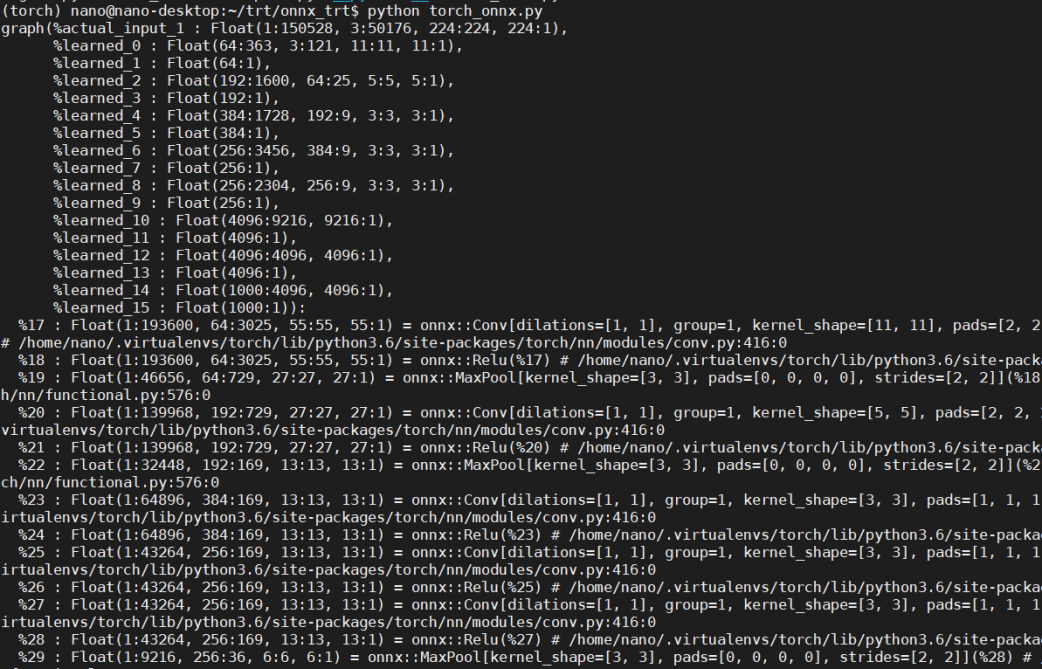The resulting alexnet.onnx is a binary protobuf file which contains both the network structure and parameters of the model you exported (in this case, AlexNet). The keyword argument verbose=True causes the exporter to print out a human-readable representation of the network:

# 檢查ONNX模型

``\$ pip install onnx``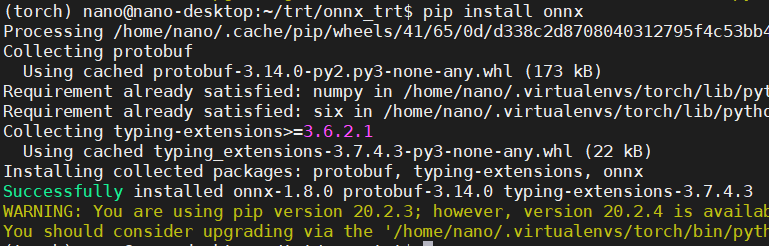``import onnx# Load the ONNX modelmodel = onnx.load("alexnet.onnx")# Check that the IR is well formedprint(onnx.checker.check_model(model))print('-'*50)# Print a human readable representation of the graphprint('Model :\n\n{}'.format(onnx.helper.printable_graph(model.graph)))``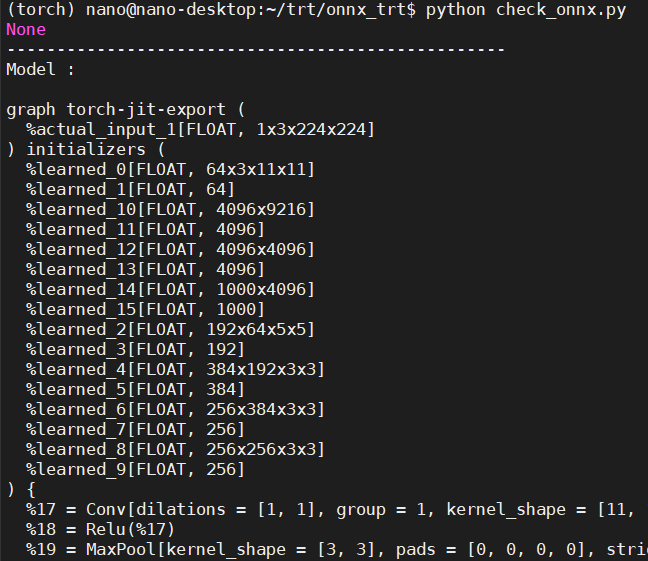## 圖形化顯示ONNX模型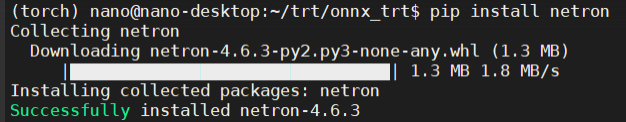``\$ netron [FILE]``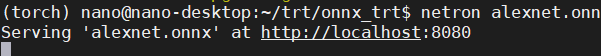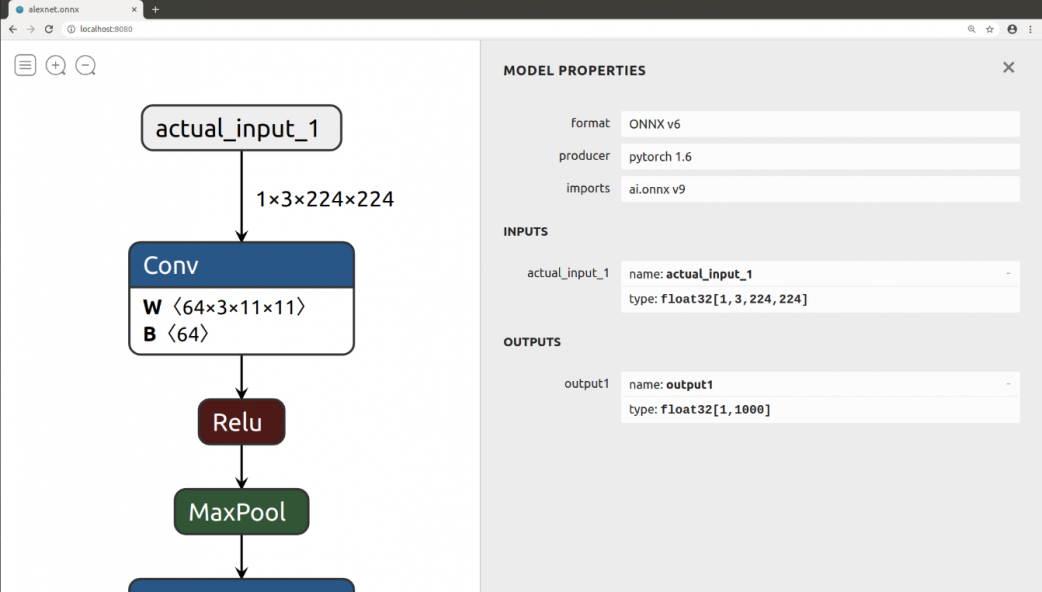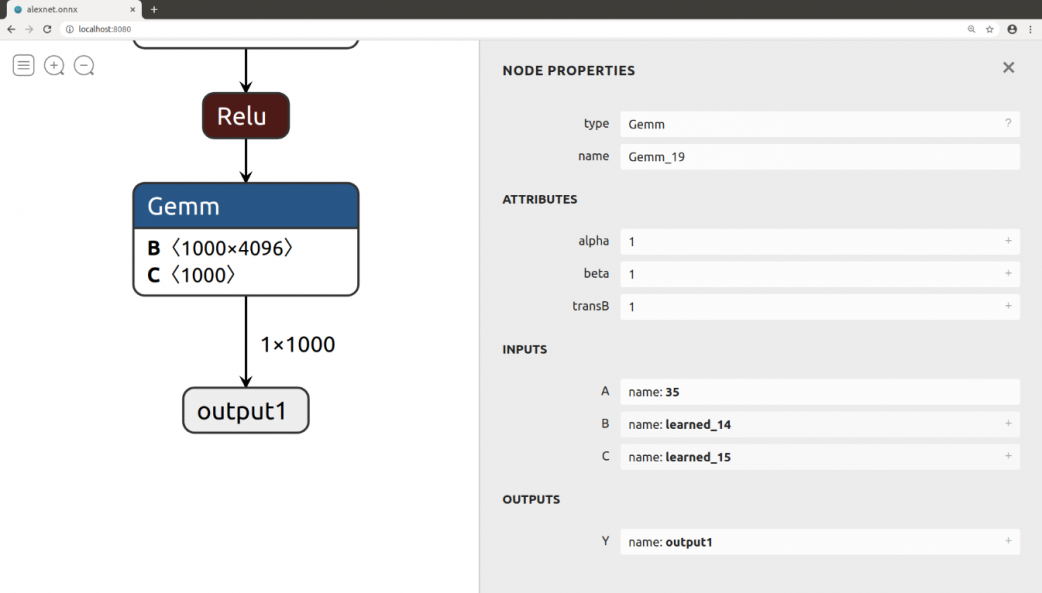# 透過ONNX RUNTIME運行ONNX Model

``\$ pip install onnxruntime``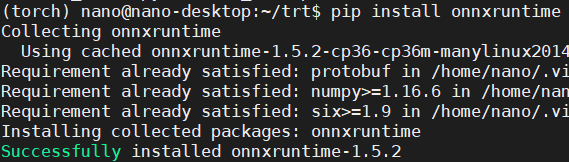``import onnxruntime as ortort_session = ort.InferenceSession('alexnet.onnx')outputs = ort_session.run(None, {'actual_input_1': np.random.randn(10, 3, 224, 224).astype(np.float32)})print(outputs)``

### ORT 運行 AlexNet

``import timedef print_div(text, custom='', end='\n\n'):    print('-'*50, '\n')    print(f"{text}{custom}", end=end)class timer():        def __init__(self, text):        print_div(text, '...', end=' ')        self.t_start = time.time()    def end(self):        t_cost = time.time() - self.t_start        print('\033[35m', end='')        print('Done ({:.5f}s)'.format(t_cost))        print('\033[0m')class logger():    def __init__(self, text):        print_div(text)``

``import onnxruntime as ortimport timefrom PIL import Imageimport numpy as npfrom torchvision import transforms as T# Custom from log import timer, logger``

``trans = T.Compose([T.Resize(256), T.CenterCrop(224), T.ToTensor()])img = Image.open('../test_photo.jpg')img_tensor = trans(img).unsqueeze(0)img_np = np.array(img_tensor)logger('Image : {} >>> {}'.format(np.shape(img) , np.shape(img_tensor)))``

``# ONNX Run Timeload_onnx = timer('Load ONNX Model')ort_session = ort.InferenceSession('alexnet.onnx')load_onnx.end()# run( out_feed, in_feed, opt )input_name = ort_session.get_inputs().nameinfer_onnx = timer('Run Infer')outputs = ort_session.run(None, {input_name: img_np})infer_onnx.end()``

``# Get Labelsf = open('../imagenet_classes.txt')t = [ i.replace('\n','') for i in f.readlines()]logger("Result : {}".format(t[np.argmax(outputs)]))``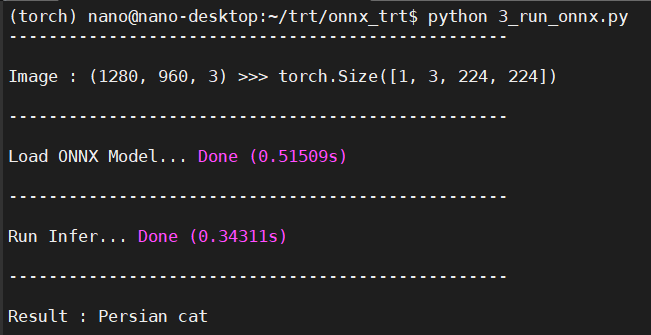### ORT運行.onnx與PyTorch運行.pth速度差異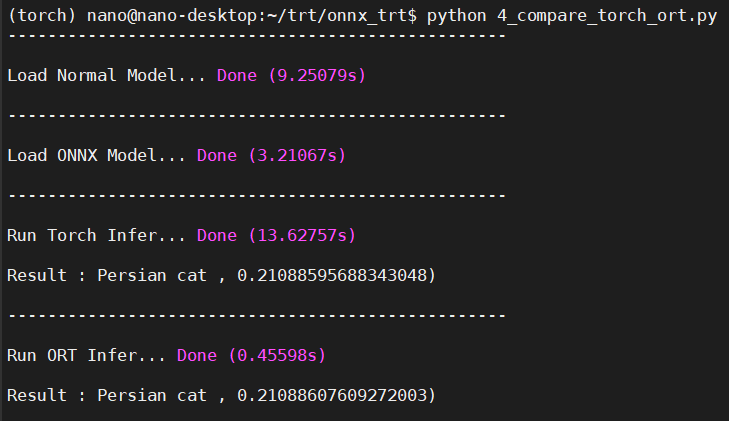#### 參考文章

*本文由RS components 贊助發表，轉載自DesignSpark部落格原文連結